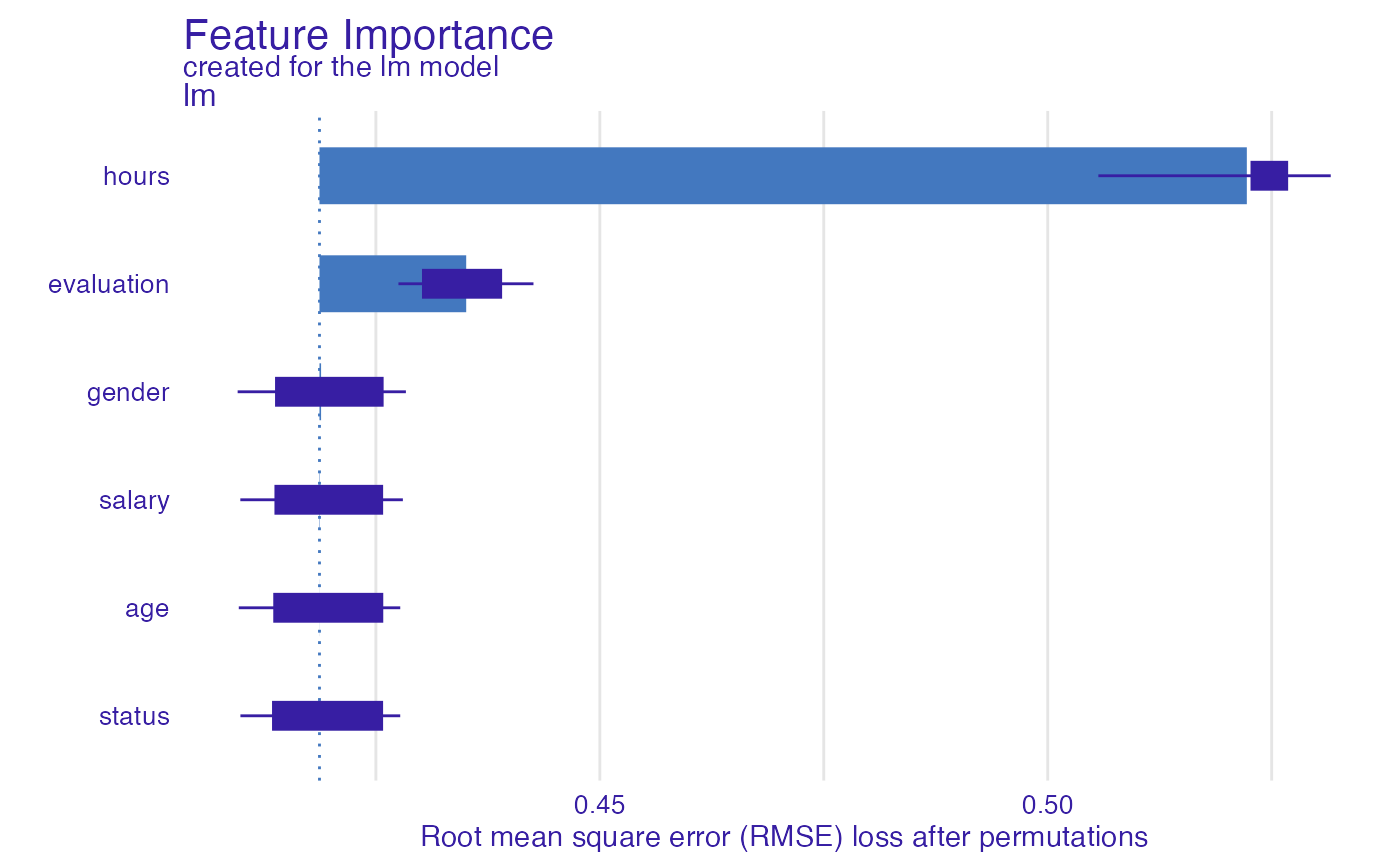This function calculates permutation based feature importance. For this reason it is also called the Variable Dropout Plot.

feature_importance(x, ...)

# S3 method for explainer
feature_importance(
x,
loss_function = DALEX::loss_root_mean_square,
...,
type = c("raw", "ratio", "difference"),
n_sample = NULL,
B = 10,
variables = NULL,
variable_groups = NULL,
N = n_sample,
label = NULL
)

# S3 method for default
feature_importance(
x,
data,
y,
predict_function = predict,
loss_function = DALEX::loss_root_mean_square,
...,
label = class(x),
type = c("raw", "ratio", "difference"),
n_sample = NULL,
B = 10,
variables = NULL,
N = n_sample,
variable_groups = NULL
)

## Arguments

x an explainer created with function DALEX::explain(), or a model to be explained. other parameters a function thet will be used to assess variable importance character, type of transformation that should be applied for dropout loss. "raw" results raw drop losses, "ratio" returns drop_loss/drop_loss_full_model while "difference" returns drop_loss - drop_loss_full_model alias for N held for backwards compatibility. number of observations that should be sampled for calculation of variable importance. integer, number of permutation rounds to perform on each variable. By default it's 10. vector of variables. If NULL then variable importance will be tested for each variable from the data separately. By default NULL list of variables names vectors. This is for testing joint variable importance. If NULL then variable importance will be tested separately for variables. By default NULL. If specified then it will override variables number of observations that should be sampled for calculation of variable importance. If NULL then variable importance will be calculated on whole dataset (no sampling). name of the model. By default it's extracted from the class attribute of the model validation dataset, will be extracted from x if it's an explainer NOTE: It is best when target variable is not present in the data true labels for data, will be extracted from x if it's an explainer predict function, will be extracted from x if it's an explainer

## Value

an object of the class feature_importance

## Details

Find more details in the Feature Importance Chapter.

Explanatory Model Analysis. Explore, Explain, and Examine Predictive Models. https://ema.drwhy.ai/

## Examples

library("DALEX")
library("ingredients")

model_titanic_glm <- glm(survived ~ gender + age + fare,
data = titanic_imputed, family = "binomial")

explain_titanic_glm <- explain(model_titanic_glm,
data = titanic_imputed[,-8],
y = titanic_imputed[,8])
#> Preparation of a new explainer is initiated
#>   -> model label       :  lm  (  default  )
#>   -> data              :  2207  rows  7  cols
#>   -> target variable   :  2207  values
#>   -> predict function  :  yhat.glm  will be used (  default  )
#>   -> predicted values  :  No value for predict function target column. (  default  )
#>   -> model_info        :  package stats , ver. 4.1.1 , task classification (  default  )
#>   -> predicted values  :  numerical, min =  0.1490412 , mean =  0.3221568 , max =  0.9878987
#>   -> residual function :  difference between y and yhat (  default  )
#>   -> residuals         :  numerical, min =  -0.8898433 , mean =  4.198546e-13 , max =  0.8448637
#>   A new explainer has been created!
fi_glm <- feature_importance(explain_titanic_glm, B = 1)
plot(fi_glm)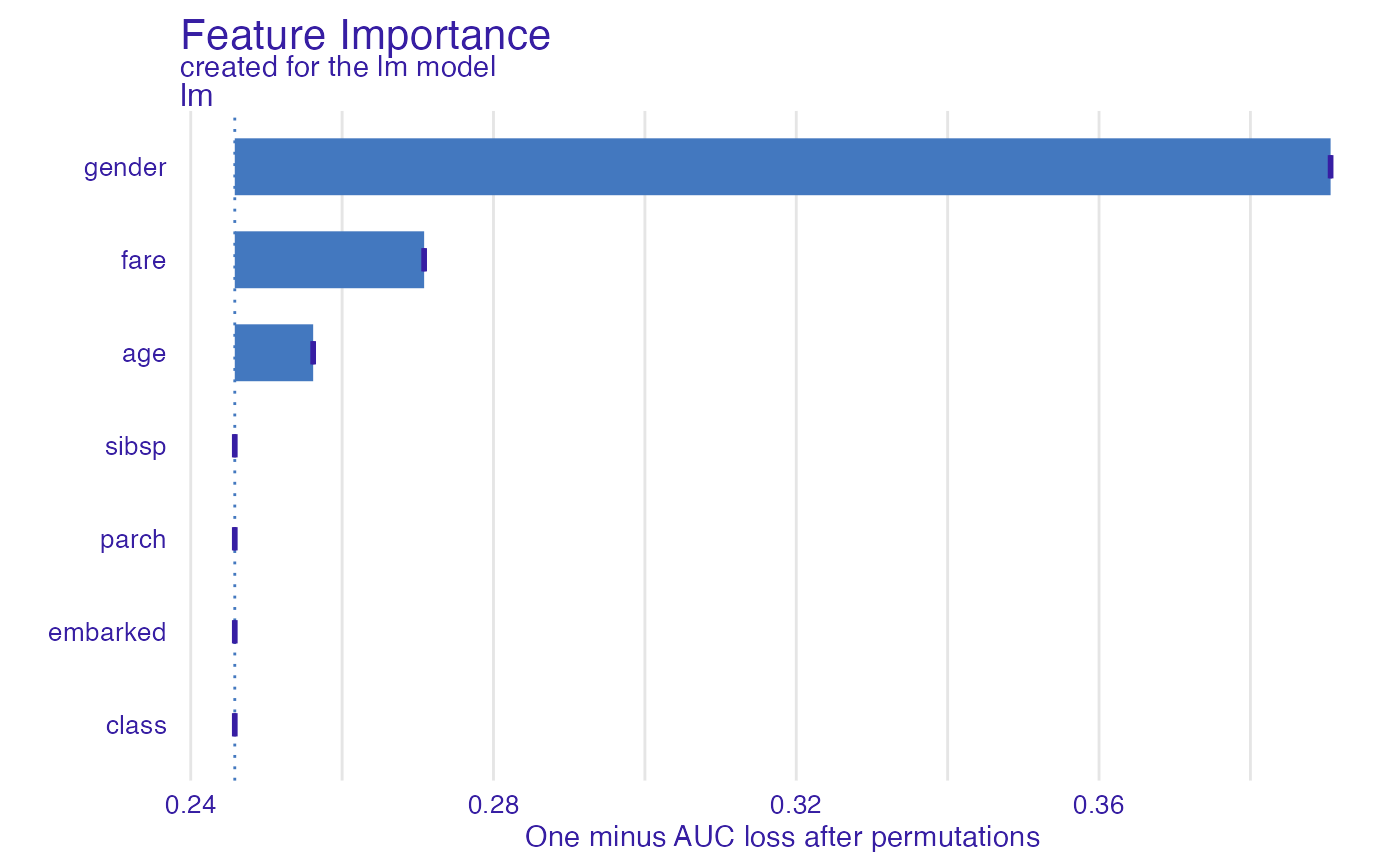# \donttest{

fi_glm_joint1 <- feature_importance(explain_titanic_glm,
variable_groups = list("demographics" = c("gender", "age"),
"ticket_type" = c("fare")),
label = "lm 2 groups")

plot(fi_glm_joint1)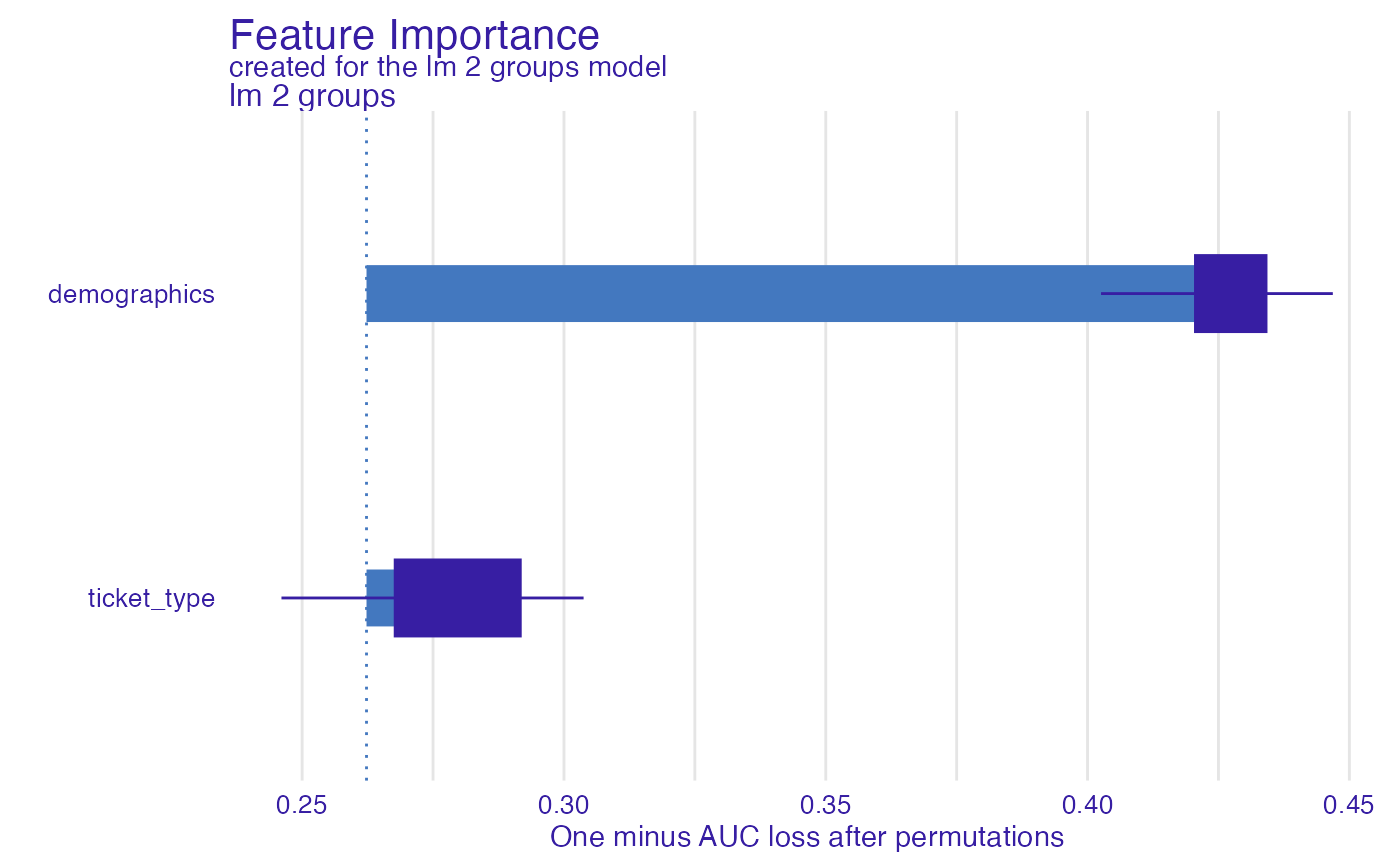fi_glm_joint2 <- feature_importance(explain_titanic_glm,
variable_groups = list("demographics" = c("gender", "age"),
"wealth" = c("fare", "class"),
"family" = c("sibsp", "parch"),
"embarked" = "embarked"),
label = "lm 5 groups")

plot(fi_glm_joint2, fi_glm_joint1)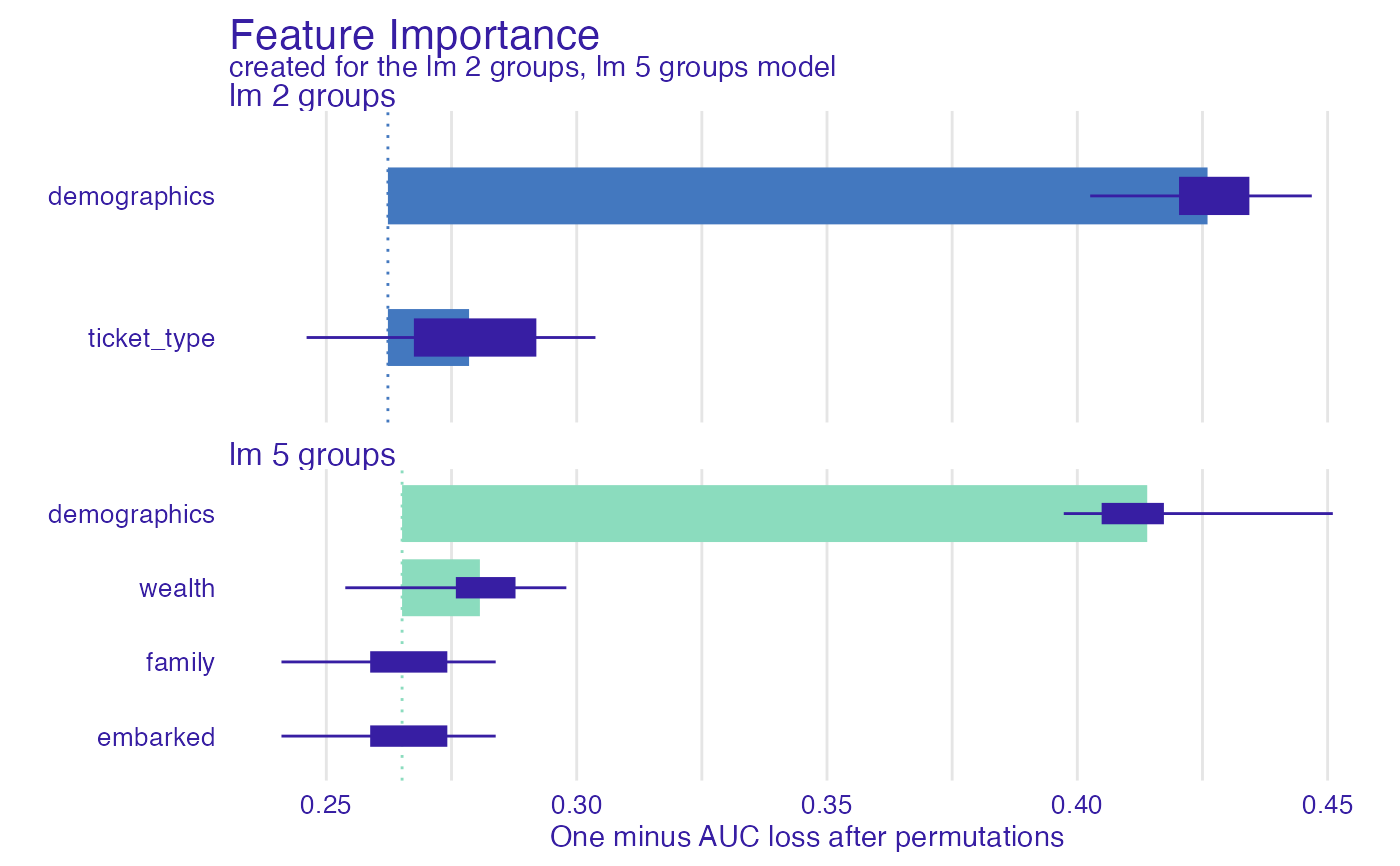library("ranger")
model_titanic_rf <- ranger(survived ~., data = titanic_imputed, probability = TRUE)

explain_titanic_rf <- explain(model_titanic_rf,
data = titanic_imputed[,-8],
y = titanic_imputed[,8],
label = "ranger forest",
verbose = FALSE)

fi_rf <- feature_importance(explain_titanic_rf)
plot(fi_rf)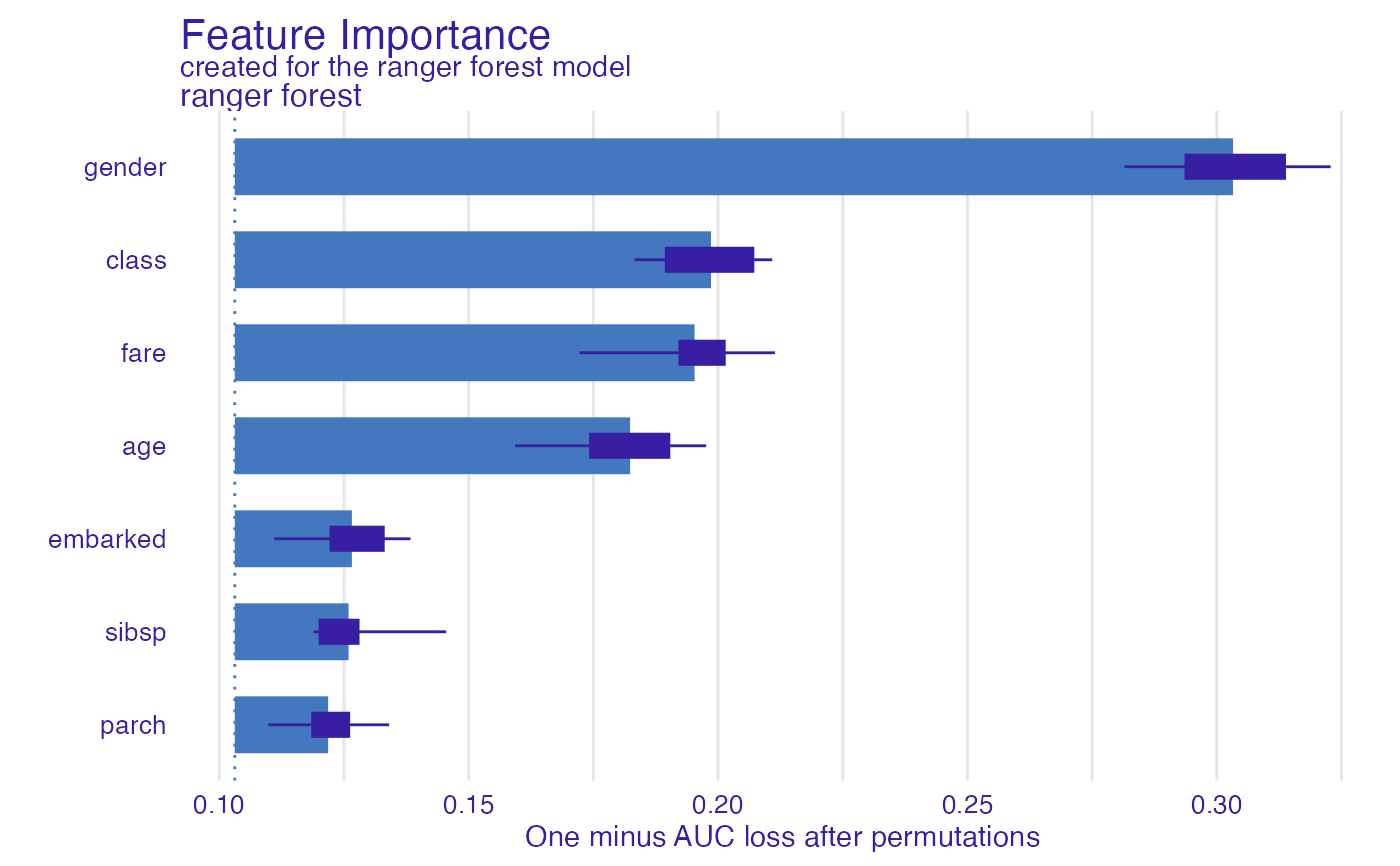fi_rf <- feature_importance(explain_titanic_rf, B = 6) # 6 replications
plot(fi_rf)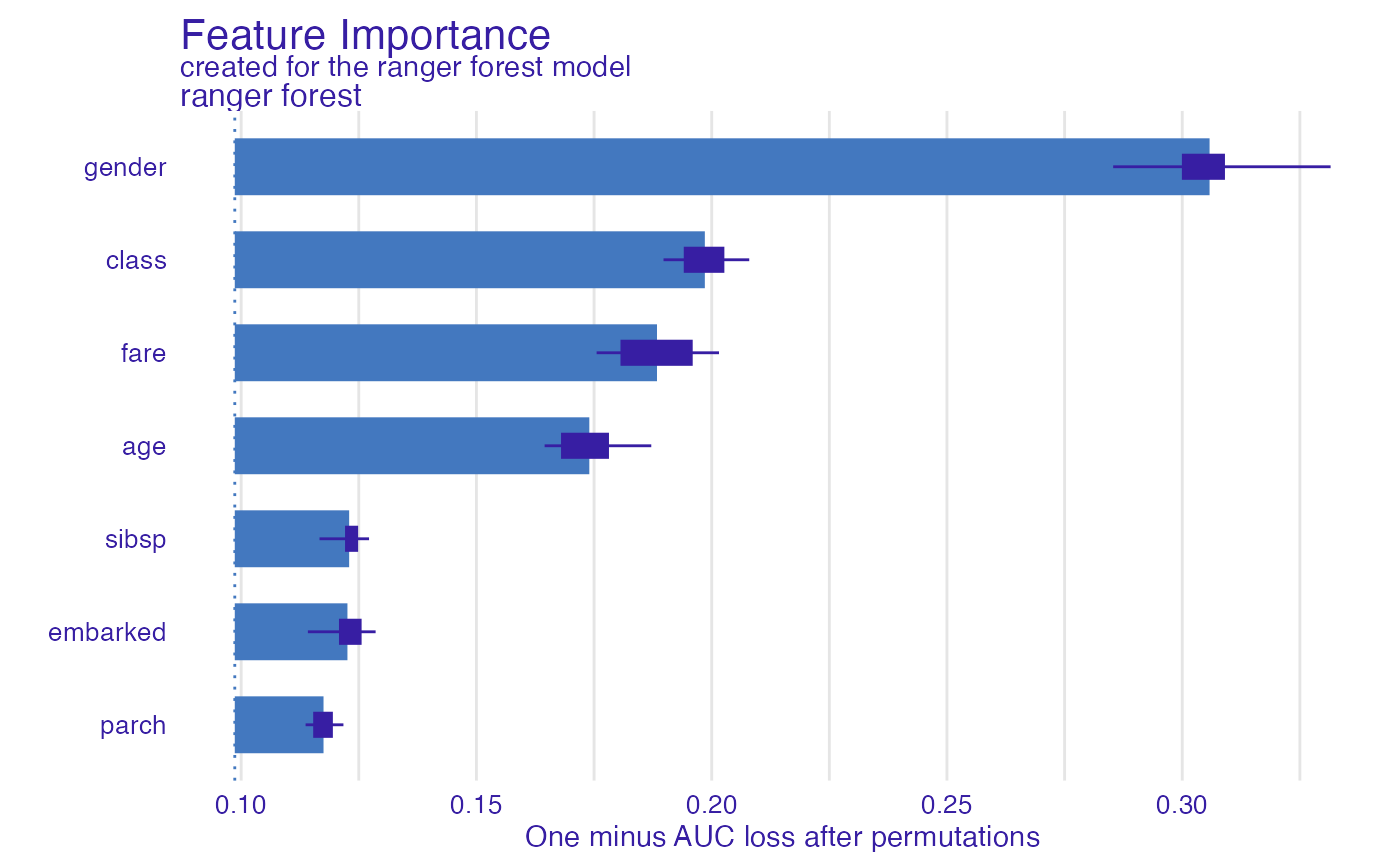fi_rf_group <- feature_importance(explain_titanic_rf,
variable_groups = list("demographics" = c("gender", "age"),
"wealth" = c("fare", "class"),
"family" = c("sibsp", "parch"),
"embarked" = "embarked"),
label = "rf 4 groups")

plot(fi_rf_group, fi_rf)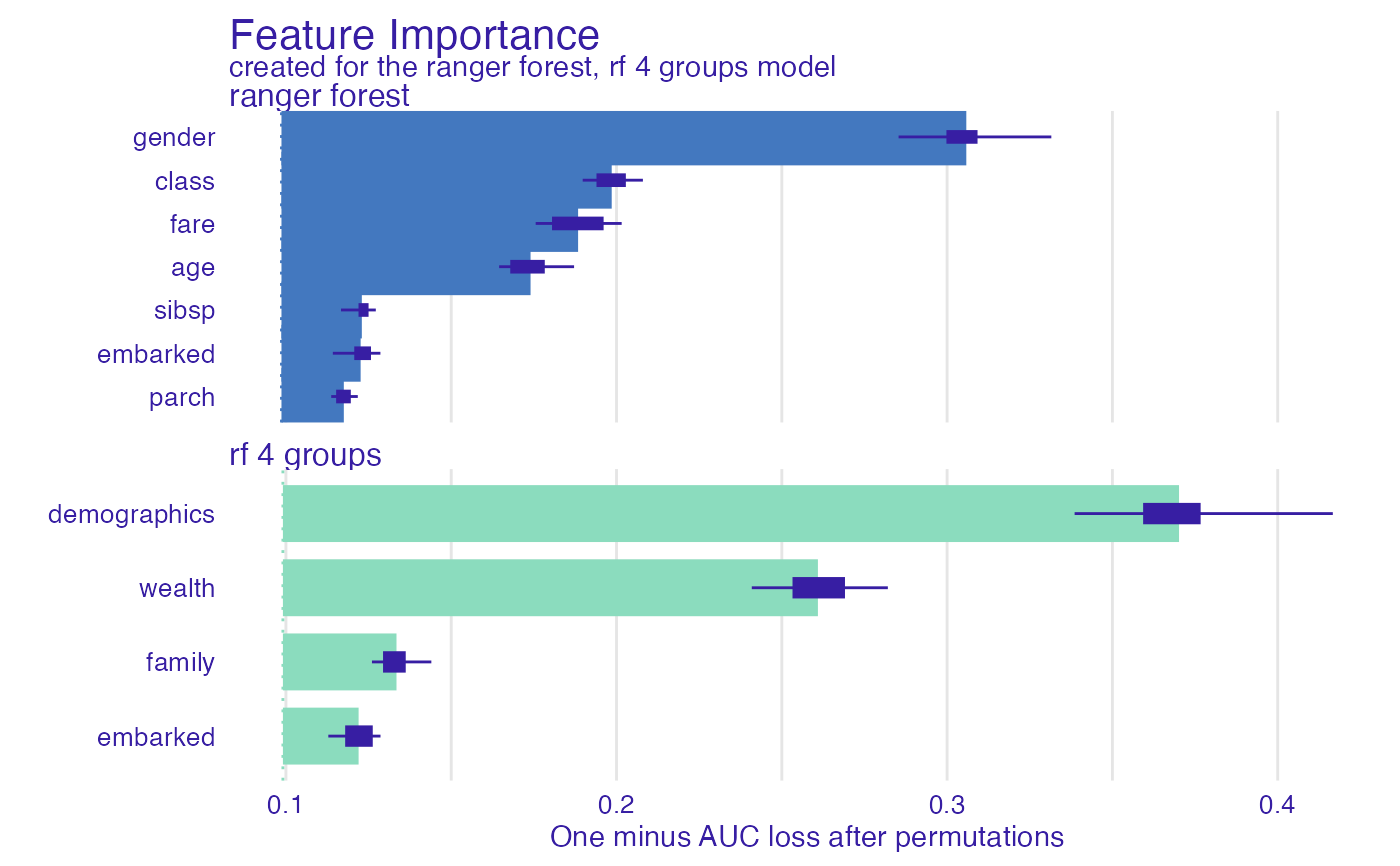HR_rf_model <- ranger(status ~., data = HR, probability = TRUE)

explainer_rf  <- explain(HR_rf_model, data = HR, y = HR$status, model_info = list(type = 'multiclass')) #> Preparation of a new explainer is initiated #> -> model label : ranger ( default ) #> -> data : 7847 rows 6 cols #> -> target variable : 7847 values #> -> predict function : yhat.ranger will be used ( default ) #> -> predicted values : No value for predict function target column. ( default ) #> -> model_info : package , ver. , task multiclass #> -> predicted values : predict function returns multiple columns: 3 ( default ) #> -> residual function : difference between 1 and probability of true class ( default ) #> -> residuals : numerical, min = 0.001843705 , mean = 0.2793549 , max = 0.8491906 #> A new explainer has been created! fi_rf <- feature_importance(explainer_rf, type = "raw", loss_function = DALEX::loss_cross_entropy) head(fi_rf) #> variable mean_dropout_loss label #> 1 _full_model_ 376.6312 ranger #> 2 status 376.6312 ranger #> 3 gender 540.6938 ranger #> 4 age 628.2120 ranger #> 5 salary 682.3624 ranger #> 6 evaluation 870.1004 rangerplot(fi_rf)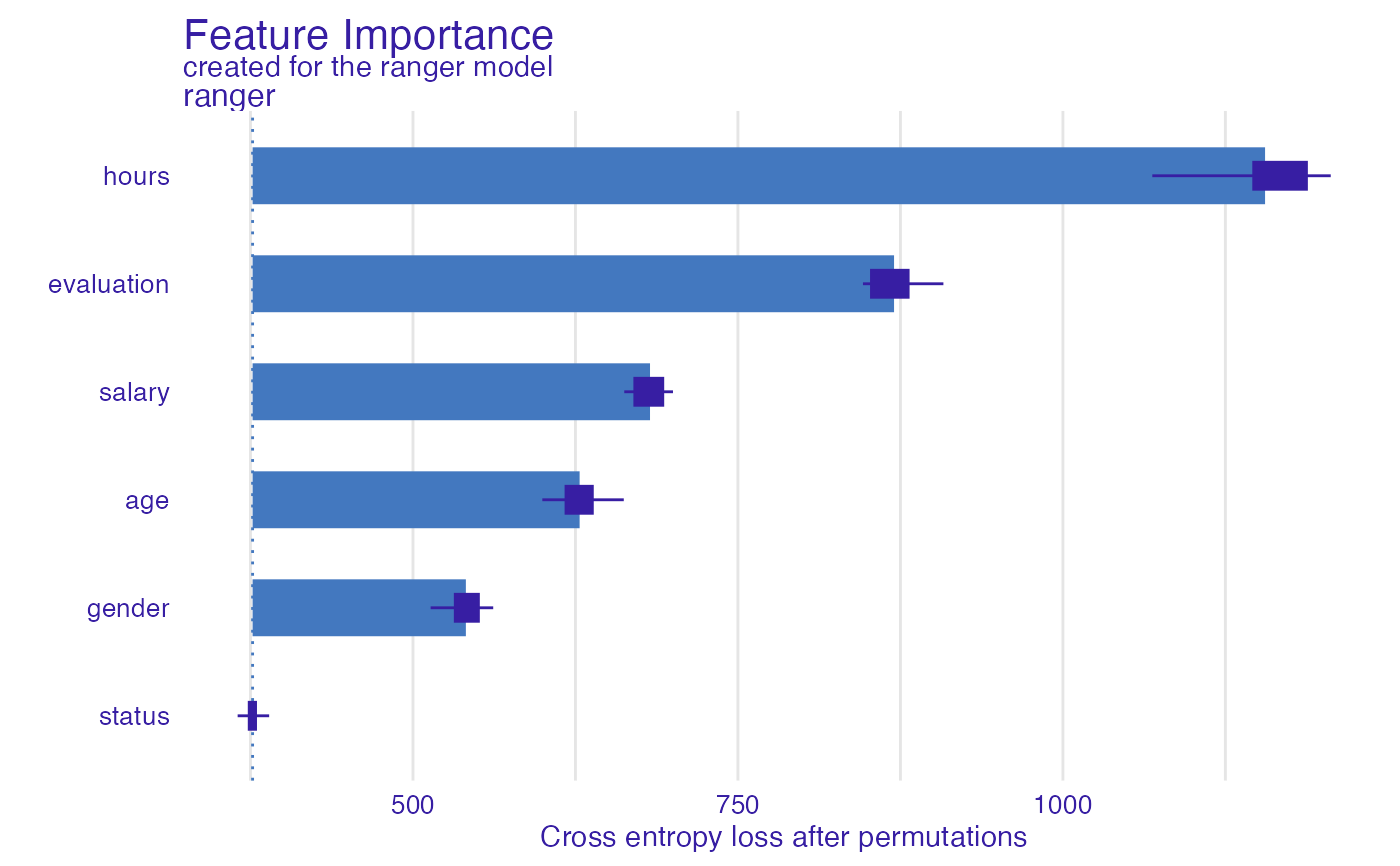HR_glm_model <- glm(status == "fired"~., data = HR, family = "binomial") explainer_glm <- explain(HR_glm_model, data = HR, y = as.numeric(HR$status == "fired"))
#> Preparation of a new explainer is initiated
#>   -> model label       :  lm  (  default  )
#>   -> data              :  7847  rows  6  cols
#>   -> target variable   :  7847  values
#>   -> predict function  :  yhat.glm  will be used (  default  )
#>   -> predicted values  :  No value for predict function target column. (  default  )
#>   -> model_info        :  package stats , ver. 4.1.1 , task classification (  default  )
#>   -> predicted values  :  numerical, min =  0.00861694 , mean =  0.3638333 , max =  0.7822214
#>   -> residual function :  difference between y and yhat (  default  )
#>   -> residuals         :  numerical, min =  -0.7755901 , mean =  -1.293796e-13 , max =  0.9820537
#>   A new explainer has been created!  fi_glm <- feature_importance(explainer_glm, type = "raw",
loss_function = DALEX::loss_root_mean_square)